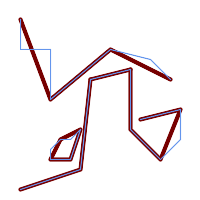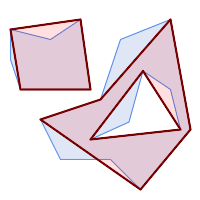## Name

ST_SimplifyPreserveTopology — Returns a simplified and valid representation of a geometry, using the Douglas-Peucker algorithm.

## Synopsis

`geometry ST_SimplifyPreserveTopology(`geometry geom, float tolerance`)`;

## Description

Computes a simplified representation of a geometry using a variant of the Douglas-Peucker algorithm which limits simplification to ensure the result has the same topology as the input. The simplification `tolerance` is a distance value, in the units of the input SRS. Simplification removes vertices which are within the tolerance distance of the simplified linework, as long as topology is preserved. The result will be valid and simple if the input is.

The function can be called with any kind of geometry (including GeometryCollections), but only line and polygon elements are simplified. For polygonal inputs, the result will have the same number of rings (shells and holes), and the rings will not cross. Ring endpoints may be simplified. For linear inputs, the result will have the same number of lines, and lines will not intersect if they did not do so in the original geometry. Endpoints of linear geometry are preserved.This function does not preserve boundaries shared between polygons. Use ST_CoverageSimplify if this is required.

Effectué par le module GEOS.

Disponibilité : 1.3.3

## Exemples

For the same example as ST_Simplify, ST_SimplifyPreserveTopology prevents oversimplification. The circle can at most become a square.

```SELECT  ST_Npoints(geom) AS np_before,
ST_NPoints(ST_SimplifyPreserveTopology(geom, 0.5)) AS np05_notquitecircle,
ST_NPoints(ST_SimplifyPreserveTopology(geom, 1))   AS np1_octagon,
ST_NPoints(ST_SimplifyPreserveTopology(geom, 10))  AS np10_square,
ST_NPoints(ST_SimplifyPreserveTopology(geom, 100)) AS np100_stillsquare
FROM (SELECT ST_Buffer('POINT(1 3)', 10,12) AS geom) AS t;

np_before | np01_notbadcircle | np05_notquitecircle | np1_octagon | np10_square | np100_stillsquare
-----------+-------------------+---------------------+-------------+-------------+-------------------
49 |                33 |                  17 |           9 |           5 |                 5
```

Simplifying a set of lines, preserving topology of non-intersecting lines.```SELECT ST_SimplifyPreserveTopology(
'MULTILINESTRING ((20 180, 20 150, 50 150, 50 100, 110 150, 150 140, 170 120), (20 10, 80 30, 90 120), (90 120, 130 130), (130 130, 130 70, 160 40, 180 60, 180 90, 140 80), (50 40, 70 40, 80 70, 70 60, 60 60, 50 50, 50 40))',
40);
```

Simplifying a MultiPolygon, preserving topology of shells and holes.```SELECT ST_SimplifyPreserveTopology(
'MULTIPOLYGON (((90 110, 80 180, 50 160, 10 170, 10 140, 20 110, 90 110)), ((40 80, 100 100, 120 160, 170 180, 190 70, 140 10, 110 40, 60 40, 40 80), (180 70, 170 110, 142.5 128.5, 128.5 77.5, 90 60, 180 70)))',
40);
```